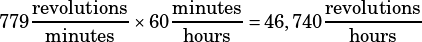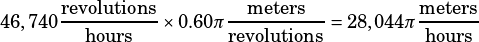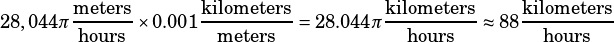An instrument shows the number of revolutions per minute made by each tire of a car. In each revolution, the car travels a distance equal to the circumference of one of its tires. The circumference of each tire is equal to 2πr, where r is the radius of the tire. If the radius of each tire on Maria’s car is 0.30 meter, what is the approximate speed of Maria’s car, to the nearest kilometer per hour, when the instrument is showing 779 revolutions per minute?

We need to do a bunch of conversions here. The order in which we do them is flexible, but I’ll convert revolutions per minute to revolutions per hour, first.From there, we can figure out how much distance the car travels in an hour by figuring out how much distance it travels in one revolution. The question tells us something which is generally useful to know for the SAT anyway: that each time a wheel makes one revolution (without slipping) it travels the distance of one circumference. In this case, a circumference of 0.30 m means each time a wheel makes one revolution the car travels.

How many meters does the car go in an hour? Well, it makes 46,740 revolutions in an hour, so:Of course, we need to answer in approximate km per hour, so let’s do one more conversion and actually multiply out that π.